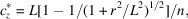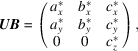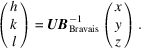International
Tables for
Crystallography
Volume C
Mathematical, physical and chemical tables
Edited by E. Prince

International Tables for Crystallography (2006). Vol. C, ch. 5.4, p. 538

## Section 5.4.1.3. Non-zero-zone analysis

A. W. S. Johnsona

#### 5.4.1.3. Non-zero-zone analysis

| top | pdf |

One pattern with well defined Laue zones taken at an arbitrary zone allows the recovery of a third vector not in the plane of the pattern. First find the components for the two shortest zero-zone vectors a* and b* (see Fig. 5.4.1.2). For the third vector, measure the x and y coordinates of a non-zero-zone vector. These are then transformed to components in the coordinate system defined by the two shortest vectors of the zero zone. Then the fractional part of each transformed component is taken. If the vector comes from a Laue zone of order n, these fractional parts must be divided by n. A transformation back to Cartesian coordinates gives the x and y components of the third vector c*, point O′ in Fig. 5.4.1.2. In practice, it is best to measure a set of vectors at roughly equal angles around the zone so that an average can be taken to improve accuracy.Figure 5.4.1.2 | top | pdf |A diffraction pattern with the crystal oriented at a zone axis. The lower diagram shows the zero-zone vectors a* and b*, and OO′, the projection on the xy plane of c*.

For certain space groups and special orientations, it is possible for half of the zero-zone reflections to be absent. If sufficient non-zero-zone vectors have been measured, two different vectors OO′ should be found. New axes (a* + b*)/2 and (a* − b*)/2 must then be chosen and the x and y coordinates of c* recalculated.

The z component of c* is obtained (Fig. 5.4.1.1) fromwhere r is the radius of the Laue circle of order n.

We now have the orientation matrix UB asand the measurement of the pattern is complete.

After transformation of the axes defined by UB to the Bravais axes, the inverse of the resulting UBBravais will index the pattern using